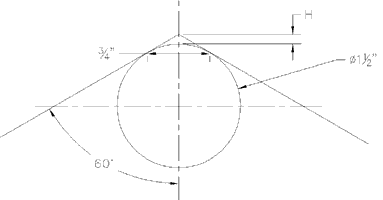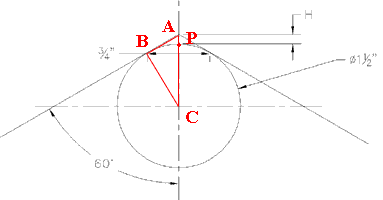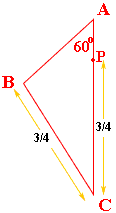Quandaries and Queries My name is Chris, I work for a custom fabricator company. I am needing a formula for the Height (H) shown in the attached picture. The picture shows dimensions for my current application. If you could please, assign variables to the dimensions. Hi Chris, Below is the diagram you sent us.If I understnd this correctly the circle has a diameter of 1.5 inches, so a radius of 0.75 inches, and the two points of tangency are 0.75 inches apart. I am going to call the center of the circle C and label three other points in the diagram below.The line CB is a radius of the circle and BA is tangent to the circle, hence the angle ABC is a right angle. The angle CAB is 60o.The sine of 60o is sqrt(3)/2 and hence |BC|/|CA| = sqrt(3)/2 and |BC| = 3/4. Thus |CA| = 3/42/sqrt(3) = sqrt(3)/2. PC is a radius of the circle so |PC| = 3/4. Thus H = |AP| = |CA| - |CP| = sqrt(3)/2 - 3/4 = (2sqrt(3) - 3)/4 which is approximately 0.1160 inches. Cheers, Harley Go to Math Central